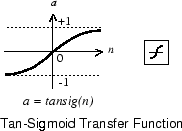# tansig

Hyperbolic tangent sigmoid transfer function

## Syntax

``A = tansig(N)``

## Description

example

``` TipTo use a hyperbolic tangent activation for deep learning, use the `tanhLayer` function or the `dlarray` method tanh. `A = tansig(N)` takes a matrix of net input vectors, `N` and returns the `S`-by-`Q` matrix, `A`, of the elements of `N` squashed into `[-1 1]`.`tansig` is a neural transfer function. Transfer functions calculate the output of a layer from its net input.```

## Examples

collapse all

This example shows how to calculate and plot the hyperbolic tangent sigmoid transfer function of an input matrix.

Create the input matrix, `n`. Then call the `tansig` function and plot the results.

```n = -5:0.1:5; a = tansig(n); plot(n,a) ```

Assign this transfer function to layer `i` of a network.

```net.layers{i}.transferFcn = 'tansig'; ```

## Input Arguments

collapse all

Net input column vectors, specified as an `S`-by-`Q` matrix.

## Output Arguments

collapse all

Output vectors, returned as an `S`-by-`Q` matrix, where each element of `N` is squashed from the interval ```[-inf inf]``` to the interval `[-1 1]` with an "S-shaped" function.

## Algorithms

```a = tansig(N) = 2/(1+exp(-2*N))-1 ```

This is mathematically equivalent to `tanh(N)`.

 Vogl, T. P., et al. ‘Accelerating the Convergence of the Back-Propagation Method’. Biological Cybernetics, vol. 59, no. 4–5, Sept. 1988, pp. 257–63. DOI.org (Crossref), doi:10.1007/BF00332914.